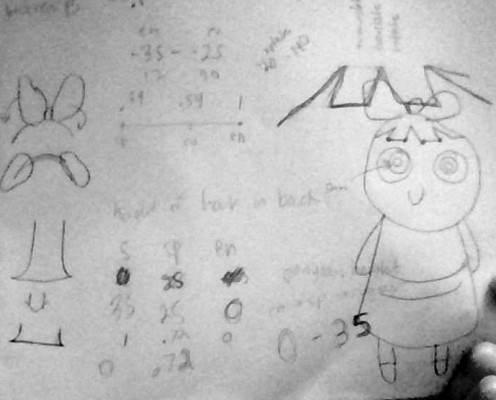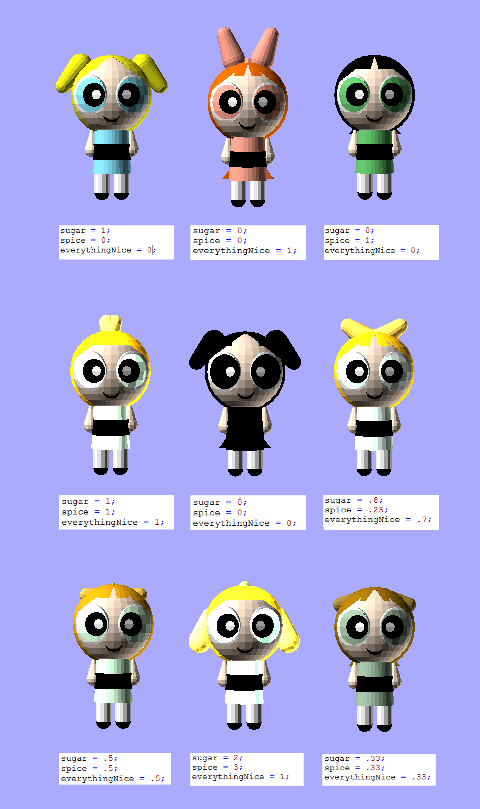ST

10 Feb 2015Parametric Powerpuffs by . Customize powerpuffs with sugar, spice, and everything nice

I came across the idea to make parametric power puff girls when I was reciting the formula. Sugar, spice, and everything nice (chemical x not included). I started thinking about changing those three variables to get all of the girls in between blossom, bubbles, and buttercup.It was certainly a challenge to figure out what parts could be reused in each of the girls. I started by modeling buttercup, and then the others, to get my initial bounds and positioning.

Overall, I am very pleased with the result. At each stage, the girls are different. It is really fun to play with and even plugging in numbers outside of the suggested range (0-1) yields interesting results.Unfortunately, OpenSCAD will not export my files to .STI because “the object isn’t a valid 2-manifold :(

Here is the code, which is also on GitHub:

////////// YOU SET THESE //////////
////////// FROM 0-1 /////////////
sugar = 1;
spice = 1;
everythingNice = 1;

///////////////////////////////

red = 1*everythingNice+.39*spice+.54*sugar;
green = .64*everythingNice+.76*spice+.91*sugar;
blue = .56*everythingNice+.42*spice+1*sugar;

hairRed = .98*everythingNice+.0*spice+1*sugar;
hairGreen = .42*everythingNice+.0*spice+.98*sugar;
hairBlue = 0*everythingNice+.0*spice+.1*sugar;

bowRed = 1*everythingNice+1*sugar;
bowGreen = .64*everythingNice+.98*sugar;
bowBlue = .56*everythingNice+.1*sugar;

ponytailHeight = (spice*.5+sugar*1+everythingNice*0)*40;
//////////// Dress ////////////////
color([red, green, blue]) cylinder(h = 40, r1 = 8, r2 = 10);

//////////// Belt ////////////////
translate([0, 0, 25])
color([0,0,0]) cylinder(h =8, r1 =9.8, r2 = 9.8);

color([1, .90, .81])sphere (r = 20);

//////////// Legs ////////////////
translate([0, 5, 30])
color([1, 1, 1]) cylinder(h =20, r1 =4 , r2 = 4);

translate([0, -5, 30])
color([1, 1, 1])cylinder(h =20, r1 =4 , r2 = 4);

///////////// Feet ///////////////
translate([0, -5, 50])
color ([0,0,0])sphere (r = 4);

translate([0, 5, 50])
color ([0,0,0])sphere (r = 4);

//////////// Arms ////////////////
translate([0, 10, 18])
color([1, .90, .81])cylinder(h =10, r1 =1 , r2 = 4);

translate([0, -10, 18])
color([1, .90, .81])cylinder(h =10, r1 =1 , r2 = 4);

translate([0, 10, 28])
color([1, .90, .81])sphere (r = 4);

translate([0, -10, 28])
color([1, .90, .81])sphere (r = 4);

// Mouth ////////////

intersection()
{
color([0,0,0])  sphere (r = 23);
difference(){
translate([13, 0, 8])
color([0,0,0]) sphere(r = 6);
translate([15, 0, 8])
color([0,0,0]) sphere(r = 5);
}}

/////////// Eyes /////////////////
intersection() {
color([1,1,1])  sphere (r = 21.5);
translate([15, 11, 0])
color([1,1,1]) sphere(r = 10);
}
intersection() {
color([1,1,1])  sphere (r = 21.5);
translate([15, -11, 0])
color([1,1,1]) sphere(r = 10);
}

color([red, green, blue]){
intersection() {
sphere (r = 22);
translate([19, 11.5, 0])
sphere(r = 9);
}
intersection() {
sphere (r = 22);
translate([19, -11.5, 0])
sphere(r = 9);
}
}

intersection() {
color([0,0,0])  sphere (r = 23);
translate([19, 8.5, 0])
color([0,0,0]) sphere(r = 6);
}
intersection() {
color([0,0,0])  sphere (r = 23);
translate([19, -8.5, 0])
color([0,0,0]) sphere(r = 6);
}

intersection() {
color([1,1,1])  sphere (r = 24);
translate([21, 7, 0])
color([0,0,0]) sphere(r = 3);
}
intersection() {
color([1,1,1])  sphere (r = 24);
translate([21, -7, 0])
color([0,0,0]) sphere(r = 3);
}

////////// Hair ///////////////

color([hairRed, hairGreen, hairBlue])intersection() {
translate([-2, 0, 0])
sphere (r = 21);
translate([-3, 0, 0])
sphere (r = 21);
}

// bangs
module leftBangs()
{
// left bubbles
polygon([[5,20],[21,21],[20,0]], convexity = N);

// blossom/buttercups
polygon([[13,7+2.5*spice],[20,5],[20,0], [13,10-(8*everythingNice+8*spice)]], convexity = N);

}

module rightBangs()
{
// left bubbles
polygon([[5,-20],[21,-21],[20,0]], convexity = N);

// blossom/buttercups
polygon([[13,-7-2.5*spice],[20,-5],[20,0], [13, -10+(8*everythingNice+8*spice)]], convexity = N);

}

intersection(){
translate([0, 0, 0])
color([hairRed, hairGreen, hairBlue])sphere (r = 21);
rotate(a=90, v=[0,1,0]) {
color([hairRed, hairGreen, hairBlue])linear_extrude(height = 20) rightBangs();
}
}

intersection(){
translate([0, 0, 0])
color([hairRed, hairGreen, hairBlue])sphere (r = 21);
rotate(a=90, v=[0,1,0]) {
color([hairRed, hairGreen, hairBlue])linear_extrude(height = 20) leftBangs();
}
}

///////// Ponytail/ Buttercup stuff //////

module ponyTail()
{
polygon([[-19,20],[-19,-20],[-10,-20], [-10,20]], convexity = N);
}

color([hairRed, hairGreen, hairBlue]){

difference(){
color([.98, .49, 0])linear_extrude(height = 45) ponyTail();

translate([-20, -35., 19-ponytailHeight])
rotate(a=90, v=[0,1,0]) {
color([.98, .49, 0])cylinder(h =20, r1 =30, r2 = 30);
}

translate([-20, 35., 19-ponytailHeight])
rotate(a=90, v=[0,1,0]) {
color([.98, .49, 0])cylinder(h =20, r1 =30, r2 = 30);
}

translate([-25, 30, 65])
rotate(a=180, v=[1,0,0]) {
color([.98, .49, 0])cube([20, 60, (25+ponytailHeight)]);
// hair grows, last value ~20-40
}

}

}

//////////// Bow ///////////////

color([bowRed, bowGreen, bowBlue]){
translate([0, 0, -5-30*everythingNice]){

translate([0, -25+13*everythingNice+10*spice, 10*spice]){
rotate(a=-140+120*everythingNice, v=[1,0,0]) {
cylinder(h =13, r1 =4 , r2 = 6);
sphere (r = 4);
translate([0, 0, 14]) color([1, .34, .26])sphere (r = 6);
}
}

translate([0, 25-13*everythingNice-10*spice, 10*spice]){
rotate(a=140-120*everythingNice, v=[1,0,0]) {
cylinder(h =13, r1 =4 , r2 = 6);
color([1, .34, .26])sphere (r = 4);
translate([0, 0, 14]) sphere (r = 6);
}
}
}
translate([0, 0, -20])
sphere(3*everythingNice);
}